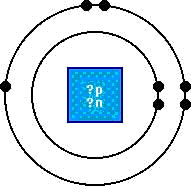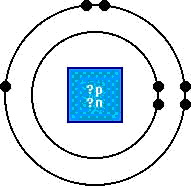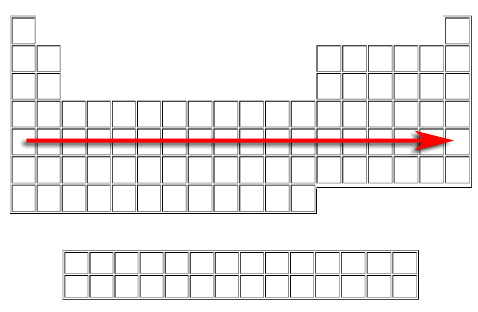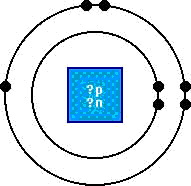Name:    Study Bank (Final Exam 2009-10)

True/False
Indicate whether the sentence or statement is true or false.

1.

Air is an element.

2.

All metals are solid at room temperature.

Multiple Choice
Identify the letter of the choice that best completes the statement or answers the question.

3.The graph shown above is typical of a
 a. boiling graph b. melting graph c. boiling or melting graph d. freeziing or melting graph e. condenstaion graph

4.

In which state of matter are molecules moving the slowest?
 a. Solid b. Liquid c. Gas d. Plasma

5.

To change a substance from a solid state to a liquid state you
 a. remove heat b. add heat c. add cold

6.

Water changes from a liquid to a gas when it
 a. melts b. loses heat c. condenses d. sublimes e. evaporates

7.

The process by whch a substance changes directly from a solid to a gas is
 a. evaporation b. sublimation c. condenstaion d. freezing e. melting

8.

Moisture that collects on a bathroom mirror during a shower is the result of what physical process?
 a. evaporation b. sublimation c. condenstaion d. freezing e. melting

9.

What process takes place when substance changes from the gaseous state to a liquid state?
 a. evaporation b. sublimation c. condenstaion d. freezing e. melting

10.

When heat is removed from a liquid its temperature goes
 a. up b. down c. remains constant

11.

At room temperature air is usually a
 a. liquid b. solid c. gas

12.

An atom of sulfur has 6 electrons in its outer shell.  It can fill its outer shell by
 a. borrowing 2 electrons b. borrowing 6 electrons c. lending2 electrons

13.

Sulfur forms
 a. positive ions b. no ions c. negative ions

14.

An atom with 2 electrons in its outer shell has a valence of
 a. -2 b. -6 c. +2

15.

Metals usually form
 a. positive ions b. no ions c. negative ions

16.

In sodium chloride, which atom completes its outer shell by borrowing an electron?
 a. sodium b. chlorine

17.

Atoms that lend electrons have a positive valence.  Atoms that borrow electrons have a negative valence.
 a. true b. false

18.

Negative ions are usually found to the right of the periodic table’s staircase.
 a. true b. false

19.

The number of electrons that an atom can lend or borrow is called its:
 a. state b. period c. phase d. valence

20.

Which of the following elements both have a valence of +1?
 a. Calcium and Magnesium b. Oxygen and Sulfur c. Potassium and Sodium d. Helium and Argon e. Fluorine and Chlorine

21.

Atoms can have their outer shells filled by gaining or losing electrons.
 a. true b. false

22.

The formula for water is H2O.  A molecule of water has
 a. 1 atom of hydrogen and 1 atom of oxygen b. 1 atom of hydrogen and 2 atoms of oxygen c. 2 atoms of hydrogen and 1 atom of oxygen

23.

A molecule of calcium chloride has
 a. 1 atom of calcium and 1 atom of chlorine b. 1 atom of calcium and 2 atoms of chlorine c. 2 atoms of calcium and 1 atom of chlorine

24.

A polyatomic ion is an electrically charged group of
 a. atoms b. molecules c. electrons

25.

An ion that is positive is
 a. sulfate b. ammonium c. nitrate

26.

How many elements are in the following compound:  (NH4)3PO4
 a. 20 b. 2 c. 4

27.

What is the valence of carbon?
 a. +4 b. +/-4 c. -4

28.

Molecules are composed of atoms.
 a. true b. false

29.

What is the name of this compound?  KClO3
 a. phosphorous chloride b. phosphorous chlorate c. potassium chloride d. potassium chlorate e. potassium bicaarbonate

30.

Water is
 a. an element b. a compound c. a mixture

31.

A gas that burns when a burning splint is held near it is
 a. oxygen b. nitrogen c. hydrogen

32.

A gas that makes a glowing splint burst into flame is
 a. oxygen b. nitriogen c. hydrogen

33.

A compound is a combination of elements.
 a. true b. false

34.

A compound is
 a. a combination of elements that cannot be easily separated b. a combination of elements that can be easily separated c. any mixture of elements that is not attracted by a magnet

35.

A compound has different properties from the elements that make it up.
 a. true b. false

36.

What is the correct formula for this compound?
Calcium+2 Hydroxide-1
 a. CaH b. CaOH c. Ca(OH) d. Ca2OH e. Ca(OH)2

37.

What is the correct formula for this compound?
Aluminum+3 Sulfate-2
 a. (NH4)2S b. (NH4)2S3 c. Al3S2 d. Al2(SO4)3 e. Al2SO4

38.

What is the correct name for this compound?
K3PO4
 a. Calcium Phosphide b. Potassium Phosphide c. Calciium Phosphate d. Potassium Phosphate e. Potassium Phosphorus Oxide

39.

When writing chemcical formulas for binary compounds, the electron donor is always written:
 a. first b. second c. last d. doesn’t matter

40.

What is the correct formula for this compound?
Lead Carbonate
 a. (Pb)(CO)3 b. (Pb)2(CO3)4 c. Pb(CO3)2 d. Ld(CO3)2 e. Pb(HCO3)2

41.

What is the correct formula for this compound?
Ammonium Bicarbonate
 a. (NH)4(HCO3) b. NH4(HCO3)1 c. NH4HCO3 d. (NH4)1(HCO3)1 e. AlHCO3

42.

A polyatomic ion is an electrically charged group of:
 a. atoms b. molecules c. electrons

43.

The formula of the hydroxide ion is
 a. OH- b. OH+ c. HO+

44.

An ion that is a positive ion is
 a. sulfate b. ammonium c. nitrate

45.

What is the maximum number electrons that can be held in the outer orbit of any atom?
 a. 2 b. 8 c. 18 d. 32 e. 103

46.The atom diagrammed above is a __?__.
 a. solid b. liquid c. gas

47.The atomic weight of the element diagrammed above is:
 a. 2 b. 5 c. 7 d. 14

48.

In neutral atoms the number of the following particles will always be equal.
 a. protons and neutrons b. neutrons and electrons c. protons and electrons

49.

The inner most orbit of any atom will never have more than __?__ electrons.
 a. 2 b. 8 c. 18 d. 32

50.

The state at which an element exists at room temperature may be determined by
 a. guessing b. the atomic number c. the number of protons d. looking at the staircase e. color code

51.

Blue symbols on the periodic table of elements represent:
 a. metals b. solids c. liquids d. gases

52.

A substance composed of two or more elements chemically combined:
 a. mixture b. solution c. precipitate d. compound

53.

3.  Which of the following represent the weight of a proton?
 a. almost nothing b. 1836 X e c. 1837 X e

54.

The nucleus of an atom contains:
 a. n & e b. n only c. p & e d. p & n

55.

.  There is a TOTAL of how many states of matter?
 a. 4 b. 3 c. 2 d. 1

56.

The nucleus of an atom has the following charge:
 a. positive b. negative c. no charge

57.

Atoms of different elements unite in chemical reactions.
 a. true b. false c. never

58.

Elements which are totally inactive are said to be:
 a. relaxed b. dead c. inert

59.

Sugar, water, and salt are all examples of:
 a. elements b. compounds c. mixtures

60.

The maximum number of electrons found in the outer orbit of an atom:
 a. 2 b. 8 c. 18 d. 32

61.

The atomic number of an element refers to the number of:
 a. electrons b. protons c. neutrons d. protrons and electrons

62.

All atoms are normally neutral.
 a. true b. false

63.

What is the maximum number of electrons the 'M' orbit can hold?
 a. 32 b. 18 c. 8 d. 2

64.

The electron dot formula shows the location of all electrons in an atom.
 a. true b. false

65.

In chemical reactions metals tend to:
 a. lose electrons b. share electrons c. gain electrons d. remain inactive

66.

When writing a chemical formula the metal is always written:
 a. first b. second c. last d. inside the parenthesis

67.

Atoms having eight electrons in their outer orbit are:
 a. positive b. negative c. inert

68.

An element having a TOTAL of 8 electrons will:
 a. gain electrons b. lose electrons c. share electrons d. do nothing

69.

Which of the following are inert:
 a. He b. Ar c. Kr d. all three

70.

H2O is the formula for one__?__of water.
 a. atom b. molecule

71.

Anything that has weight and takes up space is called:
 a. chubby b. mass c. volume d. matter

72.

Flame tests are used to identify the presence of certain:
 a. metals b. nonmetals c. compounds

73.

EVERY element we studied on the periodic table is made up of electrons, protons, and neutrons.
 a. true b. false

74.

When the 'N' orbit is the outer orbit, it is capable of holding how many electrons?
 a. 2 b. 8 c. 18 d. 32

75.

The following element has how many neutrons?   35Cl17
 a. 17 b. 18 c. 35 d. 52

76.

The following element has how many electrons?  57La139
 a. 57 b. 139 c. 82 d. 196

77.

What is the outer orbit for this element? 16S32
 a. K b. L c. M d. N

78.

The chemical symbol for sodium is:
 a. So b. SO c. Na d. na

79.

The branch of chemistry dealing with the study of carbon compounds is:
 a. biochemistry b. organic c. inorganic d. physics

80.

A branch of chemistry concerned with finding out how much of a particular substance is present is:
 a. quantitative b. qualitative c. physical d. organic

81.

The gravitational attraction on the mass of an object is:
 a. matter b. volume c. density d. weight e. mass

82.

Which of the follwing compounds is inorganic?
 a. C12H22O11 b. H2CO3 c. C10H15Cl d. Ca(BrO3)2

83.

The number of orbits an atom of an element has may be determined by its:
 a. group number b. period number c. dot formul d. atomic weight

84.

The terminals of a battery are marked + and -.  The - terminal is the:
 a. anode b. cathode c. electrode

85.

A burning splint is used to test for the presence of:
 a. oxide b. oxygen c. hydroxide d. hydrogen

86.

-------> in chemistry means:

 a. yields b. Indians! c. right turn only d. equals

87.

For each and every proton there is one neutron.
 a. true b. false

88.

Matter may be classified into:
 a. elements b. compounds c. mixtures d. all three

89.

Molecules are very close together in:
 a. solids b. liquids c. gases d. plasmas

90.

There are how many molecules shown in the formula?  6C12H22O11
 a. 3 b. 6 c. 45 d. 270

91.

There how many atoms in the formula?  2H2SO4
 a. 2 b. 7 c. 5 d. 14

92.

How many atoms of hydrogen are shown in the formula?  NH4HCO3
 a. 1 b. 2 c. 4 d. 5

93.

How many different elements are in the following compound?  HC2H3O2
 a. 1 b. 2 c. 3 d. 4

94.

Name the following radical:  HCO3
 a. hydroxide b. carbonate c. nitrate d. bicarbonate

95.

Which of the following radicals has a valence of plus one?
NH4       NO3        HCO3        ClO3
 a. chlorate b. ammonium c. nitrate d. bicarbonate

96.

What type of reaction is this?
Mg + 2HCl ------> H2 + MgCl2
 a. decomposition b. single replacement c. direct combination

97.

Is this equation balanced properly?
2NaOH + K2SO4 ------> Na2SO2 + KOH
 a. yes b. no

98.

Determine whether this atom will have a valence that is + or -.
Ag 2-8-18-18-1
 a. + b. -

99.

Determine whether this atom will have a valence that is positive or negative.
Br 2-8-18-7
 a. + b. -

100.

Which of the following represents the mass of an electron?
 a. almost nothing b. 1837 X e c. 1836 X e d. none of these

101.

Equals the number of protons plus neutrons
 a. nucleus b. period c. atomic number d. atomic weight

102.

If an element has three electrons in its outer orbit it is a nonmetal.
 a. true b. false

103.

Atoms are normally electrically neutral.
 a. true b. false

104.

Neutrons are found both within the nucleus and outside of it.
 a. true b. false

105.

Which element has the lease atomic weight?
 a. H2 b. He c. Li d. Be

106.

IDENTIFY THE CORRECT FORMULA FOR THE FOLLOWING COMPOUND:  Sodium+1Chloride-1
 a. NaCl2 b. NaClO3 c. NH4Cl d. NaCl

107.

IDENTIFY THE CORRECT FORMULA FOR THE FOLLOWING COMPOUND
Ammonium+1Bicarbonate-1
 a. NH4CO3 b. NH4(HCO3)2 c. NH4HCO3 d. NH4CO32

108.

IDENTIFY THE CORRECT FORMULA FOR THE FOLLOWING COMPOUND  Aluminum+3Oxide+2
 a. AlO b. AlO2 c. Al2O3 d. AlO3

109.

*** IDENTIFY THE FOLLOWING COMPOUND ***
NaOH
 a. Ammonium Hydroxide b. Sodium Hydride c. Sodium Hydroxide

110.

IDENTIFY THE CORRECT FORMULA FOR THE FOLLOWING COMPOUND  Sodium+1Chlorate-1
 a. NaCl b. NaClO3 c. Na2ClO3 d. Na2Cl2

111.

*** IDENTIFY THE FOLLOWING COMPOUND ***
PbO2
 a. Mercury Oxide b. Lead  Oxide c. Silver Dioxide d. Gold Oxide

112.

*** IDENTIFY THE FOLLOWING COMPOUND ***
CaCO3
 a. Calcium Chlorate b. Calcium Carbonate c. Calcium Bicarbonate

113.

Which compound was added to water to aid the flow of electric currect?
 a. NaOH b. NaHCO3 c. H2O d. C12H22O11

114.

The composition of a molecule of a substance is represented by a chemical
 a. symbol b. equation c. radical d. formula

115.

Which of the following is diatomic?
 a. Helium b. Hydrogen c. Sodium d. Phosphorus

116.

A safe gas for inflating balloons
 a. Oxygen b. Hydrogen c. Helium d. Chlorine

117.

Protons, electrons, and neutrons have their charges arranged as follows:
 a. (-,0,+) b. (+,0,-) c. (0,+,-) d. (+,-,0)

118.

A substance composed of two or more elements chemically combined:
 a. element b. compound c. mixture

119.

MIXING both hydrogen and oxygen in one test tube will produce
 a. nothing b. water c. a loud pop d. steam

120.

In which period does the element potassium belong?
 a. 1 b. 2 c. 3 d. 4

121.

A battery is marked with a plus (+) and a (-). The + is the:
 a. anode b. cathode c. commode d. mother lode

122.

H2O is the formula for one atom of water.
 a. True b. False

123.

Which state of matter has a definite volume and no definite shape?
 a. solid b. liquid c. gas d. plasma

124.

The number of electrons in the outer orbit is represented by:
 a. group number b. period number

125.

The are how many periods on the Periodic Table of the Elements?
 a. 5 b. 6 c. 7 d. 8

126.

Mercury is a liquid at room temperature. Its Central Scientific color code is:
 a. black b. blue c. red

127.

The heaviest of the subatomic particles is the
 a. electron b. protron c. neutron d. nucleus

128.

Mass and weight are the same thing.
 a. True b. False

129.

Which state of matter exists at the highest temperatures
 a. solid b. liquid c. gas d. plasma

130.

All periodic tables have the same color code.
 a. True b. False

131.

Which state of matter has a definite shape and a definite volume?
 a. solid b. liquid c. gas

132.

What is the Central Sceintific Periodoc Table’s color code for a substance having no definite shape and no definite volume?
 a. black b. blue c. red

133.

The last natural element is:
 a. man-made b. transuranium c. lawrencium d. uranium

134.

All elements gain or lose electrons and react chemically.
 a. True b. False

135.

Horizontal rows on the Perioidic Table of elements are:
 a. periods b. groups c. inert d. synthetic

136.

The separation of a compound into its component parts by using an electric
current is:
 a. electrolyte b. anode/cathode c. electrolysis d. impossible

137.

Anything that has mass and takes up space.
 a. weight b. volume c. matter d. organic e. chemistry

138.

The measure of the gravitational attraction on the mass of an object.
 a. weight b. volume c. matter d. organic e. mass

139.

The amount of space taken up by an object.
 a. weight b. mass c. matter d. volume

140.

The study of chemical processes of living organisms
 a. Organic Chemistry b. Quantitative Chemistry c. Biology d. Physcial Chemistry e. Biochemisrty

141.

The study of carbon compuunds.
 a. Chemistry b. Inorganic Chemistry c. Physical Chemistry d. Organic Chemistry e. Qualitative Chemistry

142.

A yellow burner flame on an improperly adjusted Bunsen burner would indicate that the burner fuel contains what element?
 a. H b. Li c. Be d. C e. Au

143.

The study of atomic structure
 a. Chemistry b. Inorganic Chemistry c. Physical Chemistry d. Organic Chemistry e. Qualitative Chemistry

144.

The study of substances to determine what they contain.
 a. Quantitative Chemistry b. Inorganic Chemistry c. Physical Chemistry d. Organic Chemistry e. Qualitative Chemistry

145.

The study of noncarbon compounds.
 a. Chemistry b. Inorganic Chemistry c. Physical Chemistry d. Organic Chemistry e. Qualitative Chemistry

146.

The study of substances to determine how much of a particular material is present.
 a. Quantitative Chemistry b. Inorganic Chemistry c. Physical Chemistry d. Organic Chemistry e. Qualitative Chemistry

147.

All of the following compounds contain carbon..
NaHCO3        HCl       CO2         C12H22O11
 a. True b. False

148.

There are how many known states of matter?
 a. 1 b. 2 c. 3 d. 4 e. 5

149.

Which of the following are considered to be pure substances?
 a. Elements, Compounds and Mixtures b. Compounds and Mixtures c. Mixtures and Elements d. Mixtures e. Elements and Compounds

150.

The smallest part of an element that is still the element.
 a. proton b. neutron c. electron d. atom e. compound

151.

Which of the following represents a compound?
 a. Salt and Pepper b. H2O c. Na

152.To which period does this element belong?
 a. 1 b. II c. 2 d. 7 e. 5

153.

Horizontal rows on the periodic table are called?
 a. periods b. groups c. solids

154.

Vertical columns on the periodic table are called?
 a. periods b. groups c. metals

155.

All of the following refer to which subatomic particle?
move   weigh almost nothing   found in orbit   determine group numbers
do not have a positive charge
 a. protons b. neutrons c. electrons

156.The arrow indicates which of the following on the Periodic Table?
 a. a period b. a group

157.The arrow indicates which of the following on the periodic table?
 a. a period b. a group

Numeric Response

158.

Select the link above to answer the following question.

An atom of Argon contains how many neutrons?

159.What is the atomic number for this element?

160.This element would have how many neutrons?

161.What is the maximum number of electrons an atom with only two shells may have?

162.

Select the link above to answer the following question.

The element silver is found in which period?

Matching

Identify the following polyatomic ions.
 a. CO3 e. NH4 b. ClO3 f. NO3 c. OH g. HCO3 d. PO4 h. SO4

163.

sulfate

164.

carbonate

165.

nitrate

166.

chlorate

167.

hydroxide

168.

bicarbonate

169.

phosphate

170.

ammonium

Identify the correct symbol for each of the following elements
 a. H k. K b. Li l. Ca c. He m. Mg d. Be n. N e. C o. Na f. B p. Al g. Ne q. Si h. O r. Ar i. F s. P j. S t. Cl

171.

neon

172.

potassium

173.

argon

174.

nitrogen

175.

aluminum

176.

boron

177.

lithium

178.

fluorine

179.

beryllium

180.

sulfur

181.

oxygen

182.

calcium

183.

carbon

184.

phosphorus

185.

hydrogen

186.

magnesium

187.

chlorine

188.

helium

189.

sodium

190.

silicon